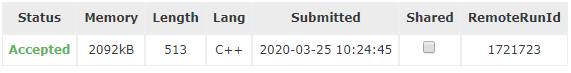# LightOJ 1265 Island of Survival 概率DP

Island of Survival LightOJ - 1265

（1）人 和 老虎：老虎吃人
（2）老虎 和 鹿：老虎吃鹿
（3）鹿 和 鹿：啥都不发生
（4）人 和 鹿：人可以选择吃或不吃鹿
（5）老虎 和 老虎：两败俱伤，都死掉

\begin{aligned} dp[i][j]&=\frac{C_i^2}{C_{i+j+1}^2}dp[i-2][j]+\frac{C_i^1C_j^1}{C_{i+j+1}^2}dp[i][j-1]+\frac{C_j^1+C_j^2}{C_{i+j+1}^2}dp[i][j]\\ dp[i][j]&=\frac{i(i-1)/2}{(i+j+1)(i+j)/2-j-j(j-1)/2}dp[i-2][j]\\ &+\frac{ij}{(i+j+1)(i+j)/2-j-j(j-1)/2}dp[i][j-1]\\ dp[i][j]&=\frac{i(i-1)}{(i+j+1)(i+j)-j-j^2}dp[i-2][j]\\ &+\frac{2ij}{(i+j+1)(i+j)-j-j^2}dp[i][j-1]\\ \end{aligned}

#include<iostream>
#include<cstdio>
//#define WINE
#define MAXN 1005
using namespace std;
int T,iCase,t,d,sum;
double dp[MAXN][MAXN],res;
int main(){
#ifdef WINE
freopen("data.in","r",stdin);
#endif
scanf("%d",&T);
while(T--){
scanf("%d%d",&t,&d);
if(t%2!=0){
printf("Case %d: 0\n",++iCase);
continue;
}
for(int i=0;i<=t;i+=2)
for(int j=0;j<=d;j++){
if(i==0){
dp[i][j]=1;
continue;
}
sum=(i+j+1)*(i+j)-j-j*j;
dp[i][j]=i*(i-1)*1.0/sum*dp[i-2][j];
if(j!=0)dp[i][j]+=2.0*i*j/sum*dp[i][j-1];
}
printf("Case %d: %.10lf\n",++iCase,dp[t][d]);
}
return 0-1;
}\begin{aligned} dp[i]&=\frac{C_i^2}{C_{i+1}^2}dp[i-2]\\ dp[i]&=\frac{i-1}{i+1}dp[i-2] \end{aligned}

#include<iostream>
#include<cstdio>
//#define WINE
#define MAXN 1005
using namespace std;
int T,iCase,t,d;
double dp[MAXN];
int main(){
#ifdef WINE
freopen("data.in","r",stdin);
#endif
scanf("%d",&T);
while(T--){
scanf("%d%d",&t,&d);
if(t%2!=0){
printf("Case %d: 0\n",++iCase);
continue;
}
dp=1;
for(int i=2;i<=t;i+=2)
dp[i]=1.0*(i-1)/(i+1)*dp[i-2];
printf("Case %d: %.8lf\n",++iCase,dp[t]);
}
return 0;
}（没写时间是因为用时 0ms…）

©️2019 CSDN 皮肤主题: 深蓝海洋 设计师: CSDN官方博客